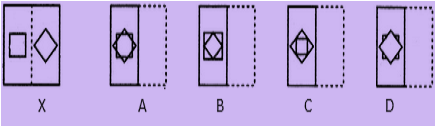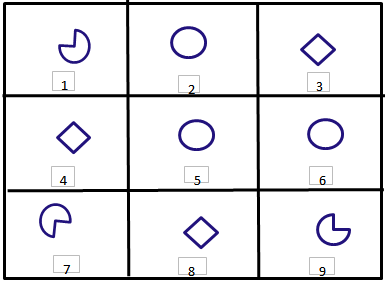# Unified Council - UIMO PDF Sample Papers for Class 9

Class 9 sample paper & practice questions for Unified International Mathematics Olympiad (UIMO) level 1 are given below. Syllabus for level 1 is also mentioned for these exams. You can refer these sample paper & quiz for preparing for the UIMO exam.#### Resources:

##### Sample Questions from Olympiad Success:
 Q.1 Q.2 Q.3 Q.4 Q.5 Q.6 Q.7 Q.8 Q.9 Q.10
 Q.1 The mean of 5 numbers is 18. If one number is excluded their mean is 16. Find the excluded number. a) 25 b) 26 c) 27 d) 28
 Q.2 Find out from the four alternatives as to how the pattern would appear when the transparent sheet is folded at the dotted line.a) A b) B c) C d) D
 Q.3 Identify the identical figures from the following options.a) (1,7,9), (2,5,6), (3,4,8) b) (1,5,9), (2,6,7), (3,4,8) c) (1,7,8), (2,5,6), (3,4,9) d) (1,2,9), (5,6,7), 3,4,8
 Q.4 If diameter of a cylinder is 5cm and volume of cylinder is 650 cm3 then height of cylinder is: a) 40 cm b) 25 cm c) 33 cm d) 45 cm
 Q.5 What is the length of the largest rod that can be put in a box of inner dimensions 30 cm, 24 cm and 18 cm? a) 20 √2 cm b) 50 cm c) 60 cm d) 30 √2 cm
 Q.6 If a cuboid is 2.7 cm high, 7.8 cm long and 3.7 cm wide then volume of cuboid is? a) 77.922 cm3 b) 82.987 cm3 c) 83.456 m3 d) 85.687 cm3
 Q.7 What is the relation between me and my paternal grandfather's son-in-law? a) brother b) maternal uncle c) maternal grandfather d) paternal uncle
 Q.8 If from the well shuffled pack of cards all the aces are removed, find the probability of getting red card. a) 1 b) 0.5 c) 1.5 d) 2
 Q.9 If we consider the normal direction format, then in which direction is Kolkata related to Madhya Pradesh? a) East b) North c) West d) South
 Q.10 A number is as much greater than 22 as is less than 72. Then the number is: a) 47 b) 50 c) 52 d) 104Sample PDF of Unified Council - Unified International Mathematics Olympiad (UIMO) PDF Sample Papers for Class 9:

 Q.1 )b Q.2 )a Q.3 )a Q.4 )c Q.5 )d Q.6 )a Q.7 )d Q.8 )b Q.9 )a Q.10 )a

Q.1 : b | Q.2 : a | Q.3 : a | Q.4 : c | Q.5 : d | Q.6 : a | Q.7 : d | Q.8 : b | Q.9 : a | Q.10 : a